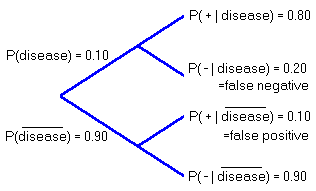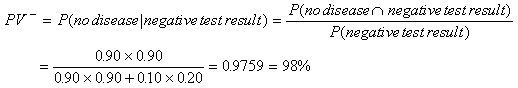# Nuclear Medicine: Planar Thallium Stress myocardial Imaging and False -Positive Test Results

Planar thallium stress myocardial imaging is a nuclear medicine diagnostic technique for predicting the presence of coronary artery disease.

Non-smoking hypertensive women in their forties with atypical chest pain have about a 10 % prevalence of significant coronary artery disease. This thallium stress test has a sensitivity of 80 % and a specificity of 90 % for coronary artery disease.

## Questions:

1. What are the false-positive and false-negative rates for thallium stress tests as defined above?
2. Determine the Predictive Value Negative for this test.

## Solution:

First some terminology:

• Specificity is the probability that the test results are negative for a person that does not have the disease.
• Sensitivity is the probability that the test results are positive for a person that does have the disease.
• Predictive Value Negative is the probability that a person does not actually have the disease if this person’s results for the test are negative.
• False Positive is the probability that a person who does not have the disease will get positive test results. In this case, the false positive rate is 10 %.
• False Negative is the probability that a person who does have the disease will get negative test results. In this case, the false negative rate is 20 %.
1. To find these rates it is helpful to draw a tree diagram:2. This is an application of Bayes’ Rule, a theorem of probability, which can be used to revise the probability a patient does or does not have the disease based on additional information being introduced ... in this case, a positive or negative result on a this test.If the test results are negative, then there is now a 98 % chance that the woman does not actually have the disease. This is a revision of the original probabilities based on the information given by the test.Written by Louise Routledge, Tuesday, June 24, 1997